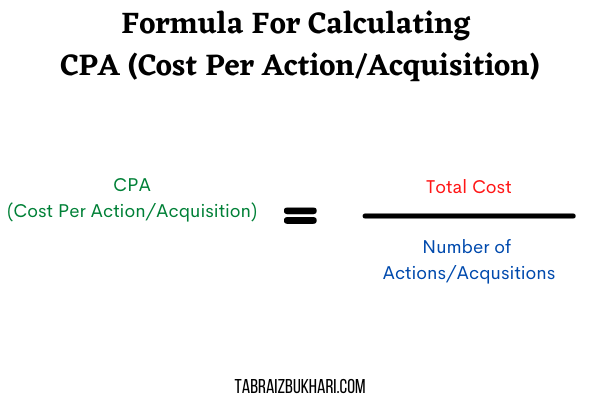CPA is short for Cost per action or cost per Acquisition. CPA tells you how much you have spent to achieve one goal (lead/conversion/sale) for your business.

Below is my calculator which tells you the CPA of your ads using the number of conversions and total cost.

## Cost Per Action Formula

The formula for calculating CPA is very easy. Cost per action is calculated by dividing the total cost by the number of actions/acquisitions completed. For example, if your ad spend was 200\$ and by spending this budget 20 actions were completed. The CPA in this situation will be 200 divided by 20 which is equal to 10.